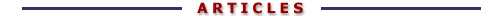## The MusArt Music-Retrieval System

### Appendix 2: Finding the Best Target Using a Query Forward Algorithm

William Birmingham, Bryon Pardo, Colin Meek, and Jonah Shifrin
Department of Electrical Engineering and Computer Science
The University of Michigan

The Forward algorithm , given an HMM and an observation sequence, returns a value between 0 and 1, indicating the probability the HMM generated the observation sequence. Given a maximum path length, T, the algorithm takes all paths through the model of up to T steps. The probability each path has of generating the observation sequence is calculated and the sum of these probabilities gives the probability that the model generated the observation sequence. This algorithm takes on the order of |S|2T steps to compute the probability, where |S| is the number of states in the model.

#### Selecting The Most Likely Model

Let there be an observation sequence (query), O, and a set of models (themes), M. An order may be imposed on M by performing the Forward algorithm on each model m in M and then ordering the set by the value returned, placing higher values before lower. The ith model in the ordered set is then the ith most likely to have generated the observation sequence. We take this rank order to be a direct measure of the relative similarity between a theme and a query. Thus, the first theme is the one most similar to the query.

We have found that, in practice, the value returned by the Forward algorithm is negatively correlated with the number of states in the model. This is an effect of model topology and the probability distribution used to determine the starting state for a model.

Many thematic models, such as that in Figure 3A, are essentially deterministic. For a deterministic model, the factors that determine the value returned by the Forward algorithm are the observation probabilities and the initial state distribution. We account for this by introducing a scaling factor that varies linearly with respect to the number of states in the model.Figure 3A: Markov model for Alouette fragment

An individual scaling factor is found for each HMM in the set of models (themes), M. When ranking themes for similarity to the observation sequence, the result of the Forward algorithm for each model is multiplied by its scaling factor and the scaled values are used to order the themes as described in the previous section.

#### Reference

 R. Durbin, S.E., A. Krogh, G. Mitchison, Biological Sequence Analysis: Probabilistic models of proteins and nucleic acids. 1998, Cambridge, UK: Cambridge University Press.

###### Copyright© 2002 William Birmingham, Bryan Pardo, Colin Meek, and Jonah Shifrin.